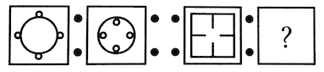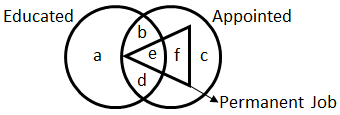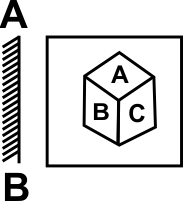﻿ Reasoning Olympiad (CRO) Previous Year Paper for Class 8

# CREST Reasoning Olympiad Class 8 Previous Year Papers

CREST Reasoning Olympiad Previous Year Paper PDF for Class 8:

## Syllabus:

Section 1: Series Completion and Inserting the Missing Character, Analogy and Classification, Blood Relations, Coding-Decoding, Direction Sense Test, Logical Venn Diagram, Alpha Numeric Sequence Puzzle, Number, Ranking and Time Sequence Test, Logical Sequence of Words, Alphabet Test, Mathematical Operations, Analytical Reasoning, Mirror Images and Water Images, Embedded Figures, Figure Formation, Construction of Squares, Grouping of Identical Figures, Figure Matrix, Paper Folding and Paper Cutting, Cubes and Dice, Dot Situations.

Achievers Section: Higher Order Thinking Questions - Syllabus as per Section 1

 Q.1 In the following question, there is a certain relationship between the two given numbers on one side of (: :) and one number is given on another side of (: :) where another number is to be found from the given alternatives, having the same relationship with this number as the numbers of the given pair has. Choose the best alternatives. 20 : 11 : : 102 : ?
 Q.2 If 'P' denotes 'multiplied by', 'T' denotes 'subtracted from', 'M' denotes 'added to' and 'B' denotes 'divided by' then, what should be the correct response of 12 P 6 M 15 T 16 B 4?
 Q.3 In the following question, select the related figures from the given alternatives.Q.4

Find the missing number in the given series.

 68 56 46 38 32 ?

 Q.5 Which of the following will replace '&' to balance the given equation? 7 & 4 & 8 & 2 = 24
 Q.6 In the following diagram, identify the region that represents appointed educated persons whose job is not permanent.Q.7 If CALANDER is coded as CLANAEDR, then CIRCULAR is coded as ____________.
 Q.8 A boy goes to see a film and finds a man who is his relative. The man is the husband of the sister of his mother. How is the man related to the boy?
 Q.9 What comes in place of the question mark in the following letter series? NATURAL, NATURA, ATURA, ?
 Q.10 In the following question, choose the correct mirror image from the alternatives, when mirror is placed on the line AB.Q.1 b Q.2 c Q.3 a Q.4 c Q.5 c Q.6 d Q.7 d Q.8 c Q.9 a Q.10 a

Here students can find the most recent CREST Reasoning Olympiad previous year paper pdf for class 8. Each question in the paper carries 1 mark. The answer key for all the questions is also provided on the last page.

Students don’t want to be left behind by their peers in the CREST Reasoning Olympiad exam, so going through the CREST Reasoning Olympiad previous year paper for class 8 is the smartest way to know how the exam paper will be. Also, practicing past year paper questions helps students quickly revise the syllabus and they get to assess their own strengths and weaknesses.